# Gaussian Elimination Calculator

Select the order of matrix, fill the required input boxes, and click the calculate button using gaussian elimination calculator

Give Us Feedback

## Gaussian Elimination Calculator

Gaussian Elimination calculator reduces a matrix formed by a system of equations to its simplified form. Find other information and values related to the matrix like:

Since this tool further solves the Gaussian elimination, it is also known as Gauss-Jordan Calculator.

## What is Gaussian elimination?

This method is named after Carl Freidrich Gauss. It uses the row echelon method to reach the desired matrix form. Then the values of the variables of the math equation are readily calculated from the resultant matrix.

It uses an augmented matrix.

## How to perform gaussian elimination?

The row operations are used on a matrix to make the diagonal entries 1 with zeros underneath. It is further used to make the matrix a diagonal matrix. This way the values are recognized from the reduced matrix itself.

First of all, you have to arrange the equation in such a way that all the same variables align column vise. If one of the equations does not contain any variable then put 0 in its place in the matrix.

Let’s see an example using this method.

### Example:

Find the values of the variables used in the following equations through the Gauss-Jordan elimination method.

1x + 1y + 2z = 9

2x + 4y - 3z = 1

3x + 6y - 5z = 0

Solution:

Step 1: Rearrange the equations to make them equal to 0.

3x + 8y - 7 = 0

6x + 3y - 3 = 0

9x + 4y - 4 = 0

Step 2: Make an augmented matrix.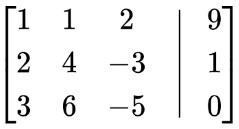Step 3: Perform row echelon reduction.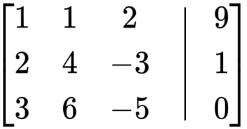Subtract row 1 multiplied by 2 from row 2: R2 = R2 - 2R1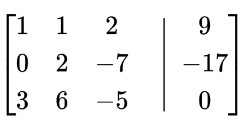Subtract row 1 multiplied by 3 from row 3: R3 = R3 - 3R1Divide row 1 by 2: R2 = R2/2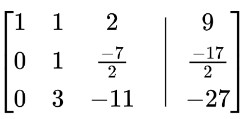Subtract row 2 multiplied by 3 from row 3: R3 = R3 - 3R2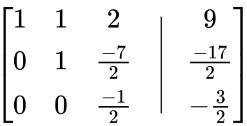Multiply row 3 by -2: R3 = -2 R3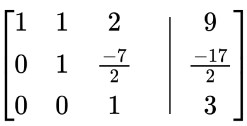This is the end of the gaussian elimination method. The values of variables can be calculated easily from this matrix. The first column represents x, 2nd is for y and 3rd shows the z variable.

There is only z in the third row. That means z=3. Put this in the second row to calculate y and after that in the first row to find x.
This matrix can be further simplified using the Gauss-Jordan method. For that:

Add row 3 multiplied by 7/3 to row 2: 7/3R3 + R2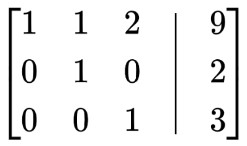Subtract row 3 multiplied by -2 from row 1: R1 = R1 -2R3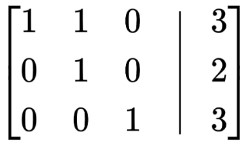Subtract row 2 from row 1: R1 = R1 - R2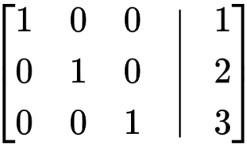From this matrix, finding the value of variables is only a matter of looking. z=3, y=2, and x=1.

### Math Tools